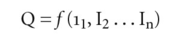# production function

Also found in: Dictionary, Thesaurus, Medical, Legal, Encyclopedia, Wikipedia.

## production function

a function that shows for a given state of technological knowledge the relationship between physical quantities of FACTOR INPUTS and the physical quantities of OUTPUT involved in producing a good or service. Since the quantity of output depends upon the quantities of inputs used, the relationship can be depicted in the form of FUNCTIONAL NOTATION:where Q = output of a product and I1 I2, etc., are quantities of the various factor inputs 1, 2, etc., used in producing that output.

It is important to emphasize that factor inputs can be combined in a number of different ways to produce the same amount of output. One method may employ large amounts of capital and only small amounts of labour, while another method may employ large quantities of labour and only a little capital. In physical terms, the method that is technically the most efficient is the one that uses the fewest inputs. Economists, however, are more concerned with the cost aspects of the input-output relationship (see COST FUNCTION), specifically the least costly way of producing a given output. Such relationships can be analysed using ISOQUANT CURVES, ISOQUANT MAPS and PROCESS RAYS.

References in periodicals archive ?
While the efficiency limit is considered as 1 in the Data Envelopment Analysis, in the Stochastic Frontier Analysis, it is determined based on production function.
Moreover, output gap is estimated with the help of five methods namely the linear trend method, quadratic trend method, HP filter, production function method, and vector autoregressive method.
Chaudhry (2009) discussed the TFP in Pakistan using the Cobb-Douglas production function (CD-PF) and trans-log production function.
The present study attempts to analyze the productivity and production function in India's manufacturing sector with particular reference to the performance of government department enterprises.
Manufacturer's production function represents the mathematical form of the relationship between the amounts of production factors used with the amount of goods which produces.
Those in the first group (located at the left side of the tree diagram) are designed to estimate a production function (or cost function) and the efficiency of individual firms, whereas SFMs in the second group (located at the right side of the diagram) are useful for the analysis of efficiency factors even though these models can be also used for efficiency estimation.
This volume proposes that the aggregate production function in economics is nothing more than the mathematical transformation of an accounting identity and that an alternative approach is needed.
The form of Cobb-Douglas stochastic production function with environmental variable can be expressed as follows:
For instance, neoclassical theory and the production function based on it focused on the law of diminishing returns and, as a result, economic growth could be explained by external factors alone.
Reallocation explains the difference between aggregate total factor productivity estimated from an aggregate production function growth and sectoral productivity growth estimated from sectoral production functions in terms of reallocation of value-added, capital, and labour.
Stochastic frontier production function is used to measure technical efficiency whereas environmental efficiency is estimated as the input-oriented technical efficiency of inputs, fertilizer and pesticide.

Site: Follow: Share:
Open / Close